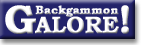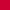TheoryForum Archive : Theory

 Derivation of drop points

 From: Michael J. Zehr Address: michaelz@michaelz.com Date: 29 April 1998 Subject: Drop point derivation (was Re: dice and backgammon variant puzzle) Forum: rec.games.backgammon Google: 3547529F.194185@michaelz.com

```Seth Breidbart wrote:
> The actual cutoff is closer to 20% chance.   (It depends on the
> position; it can be as low as 3/16, or as high as 1/4.)
>
> ObPuzzle: Give examples, and explain the 20%.
>
> Seth

There have been a couple of postings with examples, but so far no
posting giving a derivation for the 3/16, 1/4, or 1/5 figures.  The
answers build on each other, starting with the 1/4 figure, so that's
where we'll begin.  The discussion assumes no gammons and a cube
starting on 1.

Why do people usually refer to 25% as the drop point?

When you're faced with a cube, you have the choice between dropping and
taking, so you want to calculate your expected results from dropping
with the expected results (or equity) from taking and pick the most
favorable choice.

If you drop, you lose 1 point all the time, so your equity is  -1.  If
you take, and never use the cube, then if your chance of winning is p,
your equity is the equity you get if you win times the chance of
winning, plus the equity you get when you lose times the chance of
losing, or p*2 + (1-p)*-2 = 2p -2 + 2p = 4p -2.

You take the cube if this equity is greater than the equity of dropping,
so you take if:
4p-2 > -1
4p > 1
p > .25

So the drop point is 25%.  What's most important to remember about this
is that you have to win 25% of the games after taking the cube.  If
you're never going to use the cube again, then this is the same as a 25%
cubeless winning percent.  If you're going to use the cube again, then
you still need to win 25% of the games, but it isn't the same as 25%
cubeless.  (If you find  yourself in a position in which you win 85% of
the time without the cube, then you can double and win the game, not
risking those 15% losses.)

Why can you sometimes take when you win a little as 3/16ths of the time?

To answer this we first need to address the question of how winning 25%
with the cube translates to a cubeless winning percent.  To do this,
we'll use a mathematical model called "continous" backgammon.  In a
continuous game, one never goes from 65% winning chances to 80% winning
chances without at some point having a winning chance of every value in
between.  This is different from backgammon because it affects the value
of owning the cube.  If your opponent's drop point is 25%, then you gain
the most from doubling when you win 75% of the time.  If you never jump
from 65% to 80% winning chances, then you can always double at exactly
75% winning chances and get the most possible value from owning the
cube.

How do we translate 25% winning chances with the cube to a cubeless
winning chance in continuous backgammon?  We can see that if your ideal
doubling point is 75%, and your cubeless winning chances are 75%, then
your chances of winning with the cube are 100%.  You'll double, and your
opponent can drop giving you the win, or your oppponent can take, but by
the definition of the drop point, taking gives you the same expected
winnings as dropping, so no matter what your opponent does your expected
winnings in the game are as if you won the game on the current cube
level.

What if you're not exactly at the drop point?  If your ideal doubling
point is 75% and you win 25% of the time cubeless, then you're 1/3rd of
the way from losing (0%) to winning (75%), so you win 1/3rd of the
time.  (This is a result of statistical studies of something called
random walks in space.)  In general, if your cash point (100% minus your
opponent's take point) is C, and your cubeless winning percent is P,
then your winning percent with the cube is P/C.  To solve for the take
point, we set this equal to 25% (you still need to win 25% of the games
when using the dube) and solve for P, to get P = .25 * C.

Why can one sometimes take with as little as 3/16ths (about 18%) winning
chances?

If when you redouble you're giving your opponent a dead cube, then your
ideal doubling point is 75%, as we calculated above.  If you can always
reach this point exactly then the game is continuous.  As we saw above
you need to win .25 * .75, or 1/4 * 3/4 or 3/16ths of the games in order
to take the cube.

As others have already posted, there is such a position in backgammon,
where you always exactly reach your cash point and you're giving your
opponent a dead cube.  If each player has a checker on the 6 point, the
side on roll wins 75% immediately.  If they don't bear off, the other
side wins 75% of the time, so is exactly at the cash point.

Why is the take point in a continous game of backgammon equivalent to
winning 20% cubeless?

To take the cube in a continous game, you need to have a cubeless
winning percent of .25 * C, where C is the cash point.  If you're giving
your opponent a live cube, then this cash point C is equal to 1 minus
the drop point, which is .25 * C.
.25 * C = 1 - C
1.25 * C = 1
C = 1/1.25 = .80

If your cash point is 80%, your take point is .25 * 80 = 20%.

In a continous backgammon game, the drop point is 20%.  Real backgammon
games aren't continuous so you don't always get full value out of owning
the cube.  In most positions you can take with approximately 22%
cubeless winning chances.

To summarize, the take point for a dead cube is 25%, if you can always
redouble efficiently and give you opponent a dead cube, your take point
is 3/16ths or 18.75%, the take point for a live cube in a continuous
game is 20%, and in practice in real backgammon one can usually take
with 22% cubeless chances.  (All these figures still assume no gammons.)

-Michael J. Zehr
```

 Did you find the information in this article useful?           Do you have any comments you'd like to add?

### Theory

Derivation of drop points  (Michael J. Zehr, Apr 1998)
Double/take/drop rates  (Gary Wong, June 1999)
Drop rate on initial doubles  (Gary Wong, July 1998)
Error rate--Why count forced moves?  (Ian Shaw+, Apr 2009)
Error rates--Repeated ND errors  (Joe Russell+, July 2009)
Inconsistencies in how EMG equity is calculated  (Jeremy Bagai, Nov 2007)Janowski's formulas  (Joern Thyssen+, Aug 2000)
Janowski's formulas  (Stig Eide, Sept 1999)
Jump Model for money game cube decisions  (Mark Higgins+, Mar 2012)
Number of distinct positions  (Walter Trice, June 1997)
Number of no-contact positions  (Darse Billings+, Mar 2004)
Optimal strategy?  (Gary Wong, July 1998)
Proof that backgammon terminates  (Robert Koca+, May 1994)Solvability of backgammon  (Gary Wong, June 1998)
Undefined equity  (Paul Tanenbaum+, Aug 1997)Under-doubling dice  (Bill Taylor, Dec 1997)Variance reduction  (Oliver Riordan, July 2003)From GammOnLineLong messageRecommended readingRecent addition

 Book Suggestions Books Cheating Chouettes Computer Dice Cube Handling Cube Handling in Races Equipment Etiquette Extreme Gammon Fun and frustration GNU Backgammon History Jellyfish Learning Luck versus Skill Magazines & E-zines Match Archives Match Equities Match Play Match Play at 2-away/2-away Miscellaneous Opening Rolls Pip Counting Play Sites Probability and Statistics Programming Propositions Puzzles Ratings Rollouts Rules Rulings Snowie Software Source Code Strategy--Backgames Strategy--Bearing Off Strategy--Checker play Terminology Theory Tournaments Uncategorized Variations

Return to:  Backgammon Galore : Forum Archive Main Page